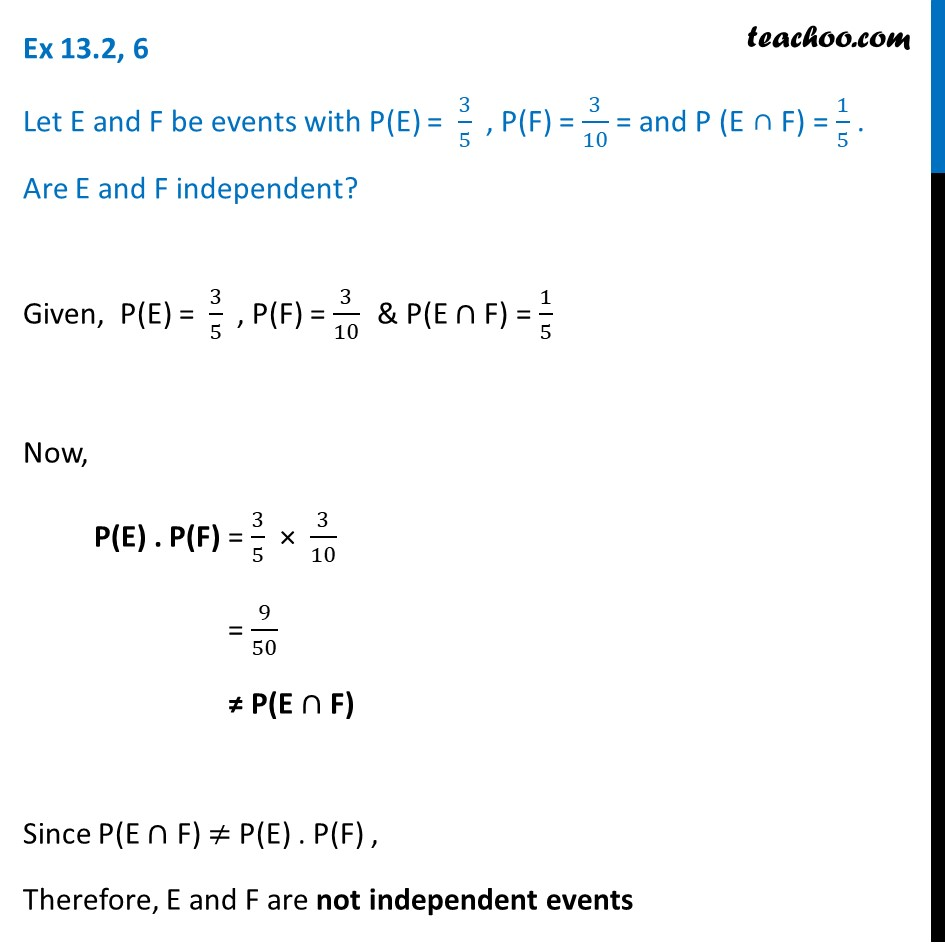Ex 13.2

Chapter 13 Class 12 Probability
Serial order wiseLearn in your speed, with individual attention - Teachoo Maths 1-on-1 Class

### Transcript

Ex 13.2, 6 Let E and F be events with P(E) = 3/5 , P(F) = 3/10 = and P (E ∩ F) = 1/5 . Are E and F independent?Given, P(E) = 3/5 , P(F) = 3/10 & P(E ∩ F) = 1/5 Now, P(E) . P(F) = 3/5 × 3/10 = 9/50 ≠ P(E ∩ F) Since P(E ∩ F) ≠ P(E) . P(F) , Therefore, E and F are not independent events